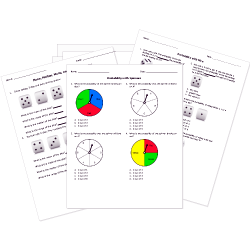Looking for Statistics worksheets?
Check out our pre-made Statistics worksheets!
 Tweet##### Browse Questions

You can create printable tests and worksheets from these Grade 10 Represent and Determine Probability questions! Select one or more questions using the checkboxes above each question. Then click the add selected questions to a test button before moving to another page.

Previous Next
Grade 10 Represent and Determine Probability CCSS: HSS-CP.A.3
Grade 10 Represent and Determine Probability CCSS: HSS-CP.A.3
Aaron is given the following situation. There are 35 students in a class, 7 of which have a 90+ grade average. He chooses two students at random, one after the other. Let choosing a student with a 90+ grade average be event A, and choosing a student with an average below a 90 be event L. He wants to know whether these events are independent or dependent. Which is it, and why? Choose all correct answers.
1. The events are dependent. After the first student has been chosen, there are fewer students, and so the probability of choosing the second student will be different than if the second student had been chosen from the full group of students.
2. The events are dependent. Since $P(L) = 4/5$, $P(L|A) = 14/17$, and these are not equal, the events are dependent.
3. The events are dependent. $P(A " then " L) = 3/85$ and $P(A) * P(L) = 4/25$. Since these are not equal, the events are not independent, and must be dependent.
4. The events are independent. Because choosing the first student as a 90+ average student doesn't change the number of students with an average below 90, the probability will remain the same regardless of the first event.
Grade 10 Represent and Determine Probability CCSS: HSS-CP.B.6
Grade 10 Represent and Determine Probability CCSS: HSS-CP.A.3
Mary is doing an experiment where she chooses two marbles, one after the other without replacement, from a bag of marbles. The bag of consists of 5 green, 6 red, and 9 blue marbles. She wants to find the probability that she chooses a blue marble given that she chose a green marble first. She lets G be the event that she chooses a green marble and B be the event that she chooses a blue marble. Mary reasons that, since $P(G) = 1/4$ and $P(B) = 9/20$, $P(G " then " B) = 9/80$. (She uses "then" instead of "and" because the events happen one after the other). Therefore, she determines that $P(B|G) = 9/20$. Is she correct, and why?
1. Yes, her assumptions and steps are all correct.
2. No, she assumed that G and B are independent when they are not. $P(G " then " B) = 9/76$, and therefore $P(B|G) = 9/19$.
3. No, she calculated P(G then B) incorrectly. $P(G " then " B) = 14/20 = 7/10$, and therefore $P(B|G) = 14/5$.
4. No, she found $P(G " then " B)$, when this is unnecessary. Since the events are independent, she can simply say $P(G) = P(G|B)$.
Grade 10 Represent and Determine Probability CCSS: HSS-CP.A.3
Adam has a bag of ten marbles, of which 3 are green, 3 are blue, and 4 are red. He also has a regular six-sided die. He lets choosing a blue marble be event A, and throwing a 5 or 6 when rolling the die be event B. Which of the following statements are correct? There may be more than one correct answer.
1. P(A) = P(A|B), since events A and B are independent.
2. P(A and B) = 0.1, since A and B are independent.
3. P(A|B) = P(B|A), since events are independent.
4. P(A|B), P(B|A) cannot be determined since not enough information has been given to determine P(A and B).
Grade 10 Represent and Determine Probability CCSS: HSS-CP.B.7
Jason and Eva are watching their friends play poker. They can see Josh's cards. Josh currently has four cards in his hand, and is about to receive his fifth and final card. He has the ace of clubs, two of clubs, three of clubs, and four of clubs. Jason and Eva agree that the best options for his next card would be the five of clubs, or any card in the clubs suit. Jason says that the probability of getting the five of clubs or any remaining club card is the same as simply the probability of getting any remaining club card. Eva disagrees, saying that the probability of simply getting any remaining club card would be different than the probability of getting any remaining club card or the five of clubs. Who is correct and why?
1. Eva is correct, because the probability of the two events must be added together, which will be higher than the probability of either event by itself since both of these events have a probability greater than zero.
2. Eva is correct, since when using the addition rule of probability, one must always add the two probabilities (in this case choosing the five of clubs and then choosing any remaining club card), and then subtract the probability that both events occur.
3. Jason is correct, because the probability of choosing the five of clubs AND any remaining club card is equal to the probability of choosing the five of clubs. Using the addition rule, these cancel out and one is left with the probability of choosing any remaining club card.
4. Jason is correct, since the probability of choosing the five of clubs is so low, that it can be ignored.
Previous Next
You need to have at least 5 reputation to vote a question down. Learn How To Earn Badges.